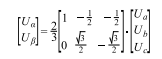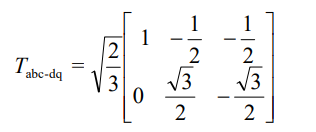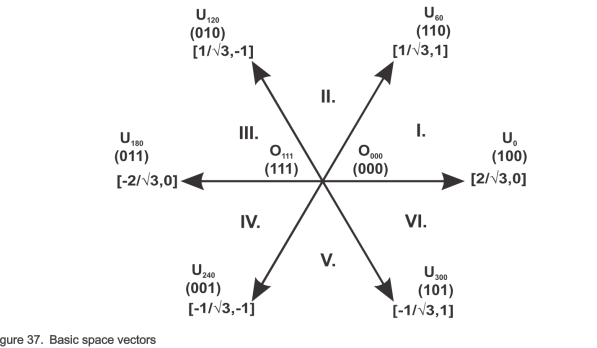# 3 phase to 2 Phase Clarke Transform

I am confused about clarke transform, since each document is using different formula, the switching pattern is

and the projection into alpha, beta as per formula is.
Here the factor is 2/3.
In one of the other TI document it is mentioned asThe factor is now Sqrt(2/3). Why is this difference?

The other question is

For the case of A =1, B=0, C=0, the UAlpha = 2Udcbus/3; UBeta = 0, but if i see the space vector diagramHere it is now 2/sqrt(3), why? If i see other document

with the factor Sqrt(2/3) the space vector is differing. Which one to follow? Lot of confusions. Can someone please help me.